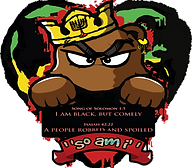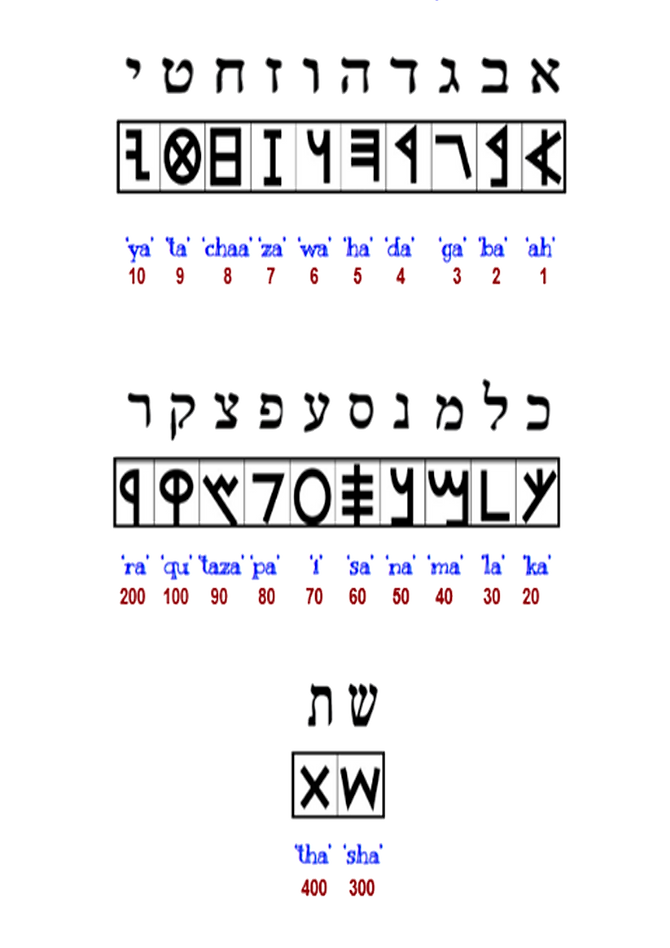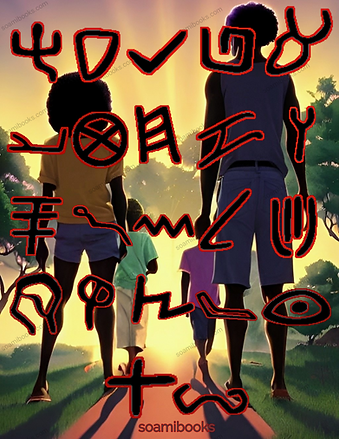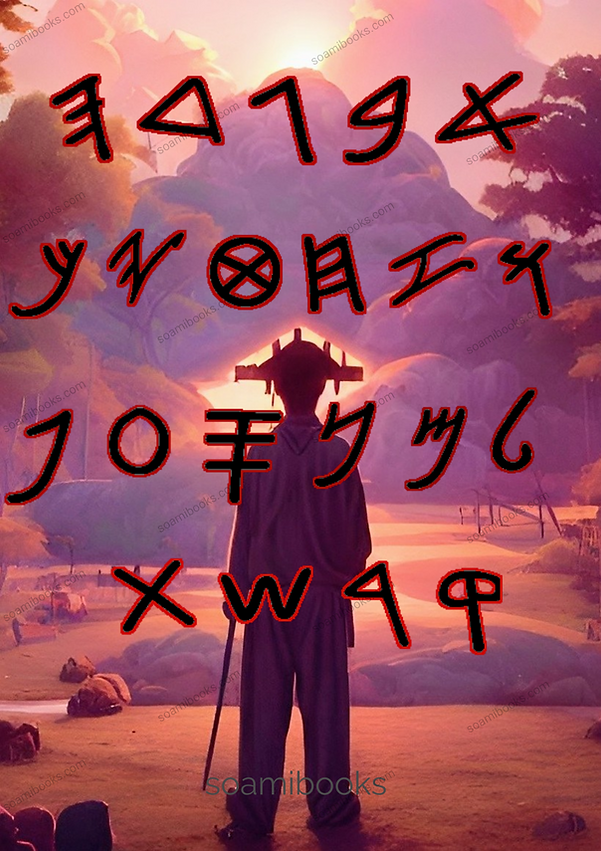top of page## Paleo Hebrew Language School## Available Now!## LEARN & PLAY PALEO HEBREW ACADEMY press 2 try now!!!## 1. YAHAWADAH = 𐤉𐤄𐤅𐤃𐤄 = יהודה = JUDAH 2. BANYAMAN = 𐤁𐤍𐤉𐤌𐤍 = בנימן = BENJAMIN 3. LAWAYA = 𐤋𐤅𐤉 = לוי = LEVI 4. SHAMAIWAN = 𐤔𐤌𐤏𐤅𐤍 = שמעון = SIMEON 5. ZABAWALAN = 𐤆𐤁𐤅𐤋𐤍 = זבולן = ZEBULON 6. AHPARYAM = 𐤀𐤐𐤓𐤉𐤌 = אפרים = EPHRAIM 7. MANASHAH =  𐤌𐤍𐤔𐤄 = מנשה = MANASSEH 8. GAD = 𐤂𐤃 = גד = GAD 9. RAAHWABAN = 𐤓𐤀𐤅𐤁𐤍 = ראובן = REUBEN 10. NAPATHALAYA = 𐤍𐤐𐤕𐤋𐤉 = נפתלי = NAPTHALI 11. AHSHAR = 𐤀𐤔𐤓 = אשר = ASHER 12. YASHASHAKAR = 𐤉𐤔𐤔𐤊𐤓 = יששכר = ISSACHAR YAWASAP =  𐤉𐤅𐤎𐤐 =יוסף = JOSEPH DAN = 𐤃𐤍 = דן = DAN YASHARAHLA =  𐤉𐤔𐤓𐤀𐤋 =  ישראל  ISRAEL AHLA SHADYA = 𐤀𐤋 𐤔𐤃𐤉 = אל שדי POWER ALMIGHTY AHLA = 𐤀𐤋 = אל  POWER AHLAHAYAM = 𐤀𐤋𐤄𐤉𐤌 = אלהים POWERS MALAK =  𐤌𐤋𐤊 = מלך  KING AHDANAYA = 𐤀𐤃𐤍𐤉 = אדני  MY LORD AHDAN = 𐤀𐤃𐤍 = אדנ LORD YARAWASHALAM = 𐤉𐤓𐤅𐤔𐤋𐤌 =ירושלם = JERUSALEM SHALAM = 𐤔𐤋𐤌 =שלם = PEACE (GREETING) BARAK AHTHAH =  𐤁𐤓𐤊 𐤀𐤕𐤄 = ברך אתה = BLESS YOU SALACHYA = 𐤎𐤋𐤇𐤉 = סלחי = FORGIVE ME SALACH = 𐤎𐤋𐤇 = סלח = FORGIVE TAWAB = 𐤈𐤅𐤁 = טוב  GOOD KAN = 𐤊𐤍 = כן = YES, SO LAAH = 𐤋𐤀 = לא  NO, NOT HALAL = 𐤄𐤋𐤋 = הלל = PRAISE HALALWAYAH =  𐤄𐤋𐤋𐤅𐤉𐤄 = הללויה = PRAISE YOU YAHAWAH, PRAISE YAHAWAH AHBA = 𐤀𐤁 = אב = FATHER AHMA = 𐤀𐤌 = אם = MOTHER BAN = 𐤁𐤍 = בן = SON BATH = 𐤁𐤕 = בת = DAUGHTER AHYASH = 𐤀𐤉𐤔 = איש = MAN AHSHAH = 𐤀𐤔𐤄 = אשה = WOMAN YALAD = 𐤉𐤋𐤃 =ילד = CHILD## LEARN & PLAY PALEO HEBREW ACADEMY

A𐤀

Abolish = Ha-Shab-Yath

About = סבב = 𐤎𐤁𐤁 = Sabab

Abundant = רב =  𐤓𐤁 = Rab

Accept = רצה = 𐤓𐤑𐤄 = Ratazah

Add = יסף = 𐤉𐤎𐤐 = Yasap

Adversary = צרר =  𐤑𐤓𐤓 = Tazarar

Affliction = Taza-Rah

Afraid = ירא = 𐤉𐤓𐤀 = Yarah

After = Ah-Chaar-Ya

Again Sha-Wab

Alarm, Warning = עוּד = 𐤏𐤅𐤃 = I-Wad

Alien, Stranger = גּיר = 𐤂𐤉𐤓 = Gayar

All = Kal

All = Kal

Alms Na-Da-Bah

Also, Too = גּם  =  𐤂𐤌 = Gam

Altar = מזבּח = 𐤌𐤆𐤁𐤇 Mazabach

Always = תּמיד = 𐤕𐤌𐤉𐤃 Thamyad

Ancient, Ancestral = זקן =  𐤆𐤒𐤍 Zaqan

Ancient = עתק = 𐤏𐤕𐤒 = Ithaq

And = Wa

And = ו = 𐤅 = Wa

Anoint=Ma-Shach

Anoint = משׁח = 𐤌𐤔𐤇 Mashach

Anointed = משׁיח =  𐤌𐤔𐤉𐤇 Mashayach

Appoint = עמד = 𐤏𐤌𐤃 Imad

Arise = Qam

Arise = קוּם = 𐤒𐤅𐤌 = Qawam

Army, Host = צבאה = 𐤑𐤁𐤀𐤄 = Tazaba-Ah

As, Like = כ = 𐤊 = Ka

Ascend =  עלה = 𐤏𐤋𐤄 I-Lah

Ask = שׁאל = 𐤔𐤀𐤋 = Sha-Al

Authority =Ma-Ra-Nath

Avenge = -Na-Qam

Awake = עוּר = 𐤏𐤅𐤓 = I-War

Away = Ra-Chaa-Naq

B𐤁

Bad Or Evil = רע = 𐤓𐤏 = Ri

Bag = Ya-La-Qa-Wat

Bag = כּלי = 𐤊𐤋𐤉 = Kalya

Battle =Qa-Rab

Be = היה = 𐤄𐤉𐤄 Hayah

Bear (Animal) = Dab

Bear = דּב = 𐤃𐤁 Dab

Beard = Za-Qa-Na

Beard = זקן = 𐤆𐤒𐤍 Zaqan

Beast =  Bah-Mah

Beast = בּהמה = 𐤁𐤄𐤌𐤄 Bahamah

Beat Ha-Kah

Beautiful = יפה = 𐤉𐤐𐤄 Yapah

Beautiful = Ya-Pah

Because = כי =  𐤊𐤉 = Kaya

Because Ka-Ya

Bed = מטּה = 𐤌𐤈𐤄 Matah

Bed Ma-Ma-Tah

Before = La-Pan-Ya

Before = קדם = 𐤒𐤃𐤌 = Qadam

Beg = Ba-Qash

Beginning = Ra-Ash-Yath Xa

Beginning = ראשׁית = 𐤓𐤀𐤔𐤉𐤕 Ra-Ashyath

Behind, After = אחר =  𐤀𐤇𐤓 = Ahchar

Behind Ah-Chaar-Ya

Believe = Ha-Ah-Ma-Yan

Believe = אמן = 𐤀𐤌𐤍 = Ahman

Below = La-Ma-Tah

Between = תּיכון = 𐤕𐤉𐤊𐤅𐤍 Thayakawan

Between Ba-Yan

Bind = Qa-Shar

Black = Sha-Chaa-War

Black = סחרת =  𐤎𐤇𐤓𐤕 = Sacharath

Blaze = La-Hab

Blaze = שׁמע =  𐤔𐤌𐤏 = Shami

Bless = בּרך = 𐤁𐤓𐤊 = Barak

Bless = Ba-Rak

Blood = דּם =  𐤃𐤌 = Dam

Blood Dam

Blue = Tha-Ka-Lath

Blue = 𐤕𐤊𐤋𐤕 = תכלת= Thakalath

Bondage = Shab-Ya

Book = Sa-Par

Book = ספר = 𐤎𐤐𐤓 = Sapar

Box = Tha-Bah

Box = תבה = 𐤕𐤁𐤄 = Thabah

Boy  = ילד = 𐤉𐤋𐤃 = Yalad

Branch = I-Nap

Branch = ענפ = 𐤏𐤍𐤐 = Inap

Brass = Na-Chaa-Shath

Brass = מחתה = 𐤍𐤇𐤕𐤄 =  Nachathah

Bread = לחם = 𐤋𐤇𐤌 = Lachaam

Break = Sha-Bar

Break = שׁבר = 𐤔𐤁𐤓 Shabar

Bring, Lead = נחה = 𐤍𐤇𐤄 = Nachah

Bring = Ba-Ya-Ah <~A

Brother = Ahch

Brother = אח = 𐤀𐤇 = Ahch

Brown = חוּם  = 𐤇𐤅𐤌 = Chawam

Brown Sha-Chaam

Bruise = Ma-Kah

Build = Ba-Nah

Build = בּנה = 𐤁𐤍𐤄  = Banah

Burn = Qa-Dach

But = Ah-Bal

Butter = Chaa-Ma-Ah

Butter = חמה = 𐤇𐤌𐤄 = Chaamah

C

Call = Qa-Ra

Call = קרא = 𐤒𐤓𐤀 = Qara

Camel = Ga-Mal

Camel = Ga-Mal

Camel = גּמל  = 𐤂𐤌𐤋 = Gamal

Captain  =Sa-Ran

Captain Sa-Ran

Captivity = Shab-Ya

Captivity = Shab-Ya

Car. Chariot = Ma-Ra-Kab A Carnal = Ba-Shar-Ya

Carnal = Ba-Shar-Ya

Carry= נשׂא = 𐤍𐤔𐤀 = Nasha

Carry Na-Sha

Cattle = בּהמה = 𐤁𐤄𐤌𐤄 = Bahamah

Cattle Ah-Lap

Celebrate = חגג = 𐤇𐤂𐤂 = Chagag

Ceremony = Ta-Kas

Chair= כּסּה = 𐤊𐤎𐤄 = Kasah

Chair Ka-Sa =

Change Sha-Nah

Chariot= מרכּבה  = 𐤌𐤓𐤊𐤁𐤄 = Marakabah

Charity=Taza-Da-Qah

Chasten Ya-Sar

Check= I-Kab

Cheese = גּבנה  = 𐤂𐤁𐤍𐤄 = Gabanah

Choose = בּחר = 𐤁𐤇𐤓 = Bachaar

Circumcise = נמל = 𐤍𐤌𐤋 = Namal

City = I-Yar

City = עיר = 𐤏𐤉𐤓 = Iyar

Clean Na-Qah

Clear Ba-Ha-Yar =

Cleave, Close= דּבק 𐤃𐤁𐤒 = Dabaq

Cleaveda-Baq

Close Sa-Gar

Cloud = ענן = 𐤏𐤁𐤍 = Iban

Cloud I-Nan

Cluster = אשׁכּול = 𐤀𐤔𐤊𐤅𐤋 = Ashkawal

Cluster Ash-Ka-Wal Coffee Qa-Pah

Cold = Qar

Cold = קר = 𐤒𐤓 = Qar

Come = בּוא =  𐤁𐤅𐤀 = Bawah

Come Ba-Ah

Comfort = Na-Chaa-Mah

Command=Taza-Wah

Command = צוה = 𐤑𐤅𐤄 = Tazawah

Communicate = Ma-Sar

Companion = Chaa-Bar

Companion = חברת = 𐤇𐤁𐤓𐤕 = Chabarath

Complete = Sha-Bi

Condemn= Pa-Sal

Conductor Chief Musician = נצח = 𐤍𐤑𐤇 = Natazach

Confidence = Ah-Ma-Wan

Conquer = פּרר =𐤐𐤓𐤓 = Parar

Conquer Ma-Na-Tazach

Consider = Chaa-Shab

Contention = Ma-Da-Wan

Contrite=I-Naw

Correction = Tha-Qa-Wan

Counselor=Ya-Wi-Taza

Counselor = יעץ = 𐤉𐤏𐤑 = Yawitaza

Courage Ah-Ma-Taza

Cow = שׁור = 𐤔𐤅𐤓 = Shawar

Cow Pa-Rah

Create = בּרא = 𐤁𐤓𐤀 = Bara

Create = Ba-Ra

Cruel = Ah-Ka-Zar

Cry = צעקה = 𐤑𐤏𐤒𐤄 = Tazaiqah

Cry Taza-Rach

Curse = Ah-Rar

Curse = ארר = 𐤀𐤓𐤓 = Ahrar

Cut = Ka-Rath

Cut = כּרת = 𐤊𐤓𐤕 = Karath

D𐤃

Damage = חבל = 𐤇𐤁𐤋 = Chaabal

Dark, Black = קדר = 𐤒𐤃𐤓 = Qadar

Darkness = Chaa-Shak

Darkness=  חשכ = 𐤇𐤔𐤊 = Chaashak

Daughter = Ba-Nath

Daughter = בּת = 𐤁𐤕 = Bath

Day = Ya-Wam

Day = יום = 𐤉𐤅𐤌 = Yawam

Deal = Sa-Chaar

Death = Ma-Ya-Thah

Debt = Chaa-Wab

Death = מות = 𐤌𐤅𐤕 = Mawath

Debate, = ריב =  𐤓𐤉𐤁 = Rayab

Debts = Chaa-Wab-Wath

Debts = משּׁאה = 𐤌𐤔𐤀𐤄 = Mashaah

Deceit Ma-Ra-Mah

Declare = Ah-Mar

Declare = בּטח = 𐤁𐤈𐤇 = Batacha

Deep = תּהום = 𐤕𐤄𐤅𐤌 = Thahawam

Deep Tha-Ha-Wam

Defend = Ha-Gan

Delight  = ענג = 𐤏𐤍𐤂 = Inag

Deliver = Ha-Taza-Yal

Deliver = שׁוּב = 𐤔𐤅𐤁 = Shawab

Depart I-Zab

Derision=" Taza-Chaa-Waq

Deserve Za-Kah Desire = Ra-Tazah

Desolate = Ha-Chaar-Yab

Destitute = עזב = 𐤏𐤆𐤁 = Izab

Destroy = אבד = 𐤀𐤁𐤃 = Ahbad

Different = Na-Ba-Dal

Diligent = Chaa-Raw-Taza

Diligent = חפשׂ = 𐤇𐤐𐤔 = Chapash

Discipline Ma-Sha-Mi

Disease = Ma-Chaa-Lah

Divide = בּדל = 𐤁𐤃𐤋 = Badal

Divide Pa-Lag

Do= Da

Do = עשׂה =  𐤏𐤔𐤄 = Ishah

Dog = כּלב = 𐤊𐤋𐤁 = Kalab

Doing = Da-Wa

Dominion = Ma-Mash-Lah

Door, Gates, Leaves, Lid = דּלת = 𐤃𐤋𐤕 = Dalath

Door = Da-Lath

Double = Ka-Pa-Wal

Doubt = Sa-Paq

Down = ירד = 𐤉𐤓𐤃 = Yarad

Down = Ma-Tah

Dream = חלם = 𐤇𐤋𐤌 = Chaalam

Drink = שׁקה = 𐤔𐤒𐤄 = Shaquah

Drunk = שׁתה = 𐤔𐤕𐤄 = Shathah

Dry = יבּשׂה = 𐤉𐤁𐤔𐤄 = Yabashah

E

Ear= Ahzan

Ear = Ah-Zan

Early= Shakam

Earring= Nazam

Earth = Ah-Ra-Taza

Earthquake= Raish

East = Qa-Dam

Eat= Ahkal

Ebony= Haban

Eden = I-Dan

Edge= Qatazah

Edom= Ahdawam

Elect= Bachaayar

Embalm= Chaanat

Embrace= Chaabag

Embroider= Shabataza

Emerald= Napak

Eminent= Thala

Empty= Napah

End=Qa-Tazah

Endure= Yakal

Enemy= Ahyabya

Enemy Ah-Yab-Ya

Enquire= Darash

Envy = Qa-Na

Establish Ha-Qa-Yam

Evening= Irab

Evil = Ri

Exalt=Ha-Ra-Yam

Extend = Ma-Thach

Eye= Iyan

Eye = I-Yan

F

Face= Panya

Face = Pan-Ya

Faith= Ahmawan

Faith Ah-Ma-Wan

Fall= Mawat

False Ka-Zab

Family = Ma-Shap-Chaah

Famine = Ra-Ib

Fast Ma-Ha-Yar

Father= Ahba

Father Ah-Ba

Fault Ma-Wam

Fear= Yaraah

Fear Ya-Ra

Feast= Chaag

Female= Naqabah

Female = Na-Qa-Bah Field = Sha-Dah

Fight= Lachaama

Fight=La-Chaa-Ma

Fill = Ma-La

Finish= Gamar

Finish = Ga-Mar

Fire= Ahsh

First= Raashwan

First Ra-Ash-Wan

Fish= Dag

Flesh Ba-Shar

Flower = Parach

Fly= Ip

Foot=Ra-Gal

Forget Na-Shah

Forgive, Excuse=Sa-Lach

Fork Ma-Za-Lag

Forsake = Za-Nach

Fowl= Iwap

Free Chaa-Pash-Ya

Friend(M Only) = Ra-I

Fringe Taza-Ya-Zah

From Ma-Yan

Fruit = Par-Ya

Full = Sha-Bi

G𐤂

Gain= Thabawaah

Gap= Parataza

Garden= Gan

Garrison= Natazayab

Gather= Qawah

Gather = Qa-Wah

Genealogy= Yachaash

Generation= Dawar

Get = Lak

Girdle = Ah-Ba-Nat

Girl Ya-La-Dah

Give = Na-Than

Give-To-Me=Na-Than-La-Ya

Glass, Cup= Kawas

Glass Ka-Was

Glutton Ba-Li- Na

Go= Yalak

Goblet= Ahgan

Gods, Powers= Ahlayam

Gods, Powers = Ah-La-Yam

Good= Tawab

Good Ta-Wab

Government-Shal

Grace= Chaan

Grape = I -Nab

Grape= Ishab

Grass I -Shab

Grateful Ah-Sa-Yar

Great Ga-Dal

Green= Yaraqa

Green = Ya-Ra-Qa

Groan = Ha-Ma-Yah

Grow= Tazamach

Grow = Taza-Mach

H𐤄

Hair= Shair

Hair = Sha-Ir

Half= Chaatazaya

Happy = Ah-Shar

Harm = Ha-Ri

Harvest= Qatazayar

Harvest = Qa-Taza-Yar

Hate = Ah-Ya-Bah

Heal = Ra-Pa

Hear, Listen= Shami

Hear, Listen Sha-Mi

Heart = Lab

Heaven = Sha-Ma-Yam

Hebrew (Language)= I-Bar-Yath

Hebrite = I-Bar-Ya

Hedge = Sa-Yag

Here = Ka-An

Hide = I-War

Hide= Kasah

High, Exalted= Ram

High = Ram

Home= Bayath

Home = Ba-Ya-Tha

Honey= Dabash

Honey Da-Bash

Hook = Wa-Wa

Hot = Chaam

Hour = Sha-I -Ha

House = Ba-Yath

Hover, Moved= Marachaapath

How = Ah-Yak

Humble = Ha-Dab-Yar

Hungry = Ra-I’ba

Hurt = Ha-Za-Yaq

Idle= Ramayah

I𐤏

If, Whether = Am

Ignorance= Shagagah

Illness Ma-Tha-Lah

Image, Idol,

Imagine= Chaashab

Impudent= Matazach

In = Ba

Incense= Qatar

Incline = Ma-Dar-Wan

Increase= Thabawaah

Increase = Tha-Was-Path

Indeed= Ahmanah

Indignation= Qatazap

Inform= Yarah

Inhabit= Shakan

Inherit= Nachaalah

Iniquities= Iwan

Innocent= Naqaya

Instruction= Masar

Instrument= Kalaya

Integrity= Tham

Intercession= Pagi

Interpret= Pathar

Iron= Barazal

Iron = Ba-Ra-Zal

Irrigate= Shaqah

Irrigate = Sha-Qah It-Ha-Wa-Ah

Israel= Yasharahla

It= Hawaah

J

Jacob= Yaiqab

Japheth= Yapath

Jasper= Yashapah

Javelin= Ramach

Jaw= Lachaaya

Jealous= Qanaah

Jehoshophat= Yahawashapat

Jeremiah= Yaramyah

Jerusalem= Yarawshalam

Jeshurun= Yasharwan

Jesse= Yashaya

Jewel= Kalaya

Jezreel= Yazariahla

Job= Ahyawab

Join= Lawah

Jonathan= Yawanathan

Joseph= Yawasap

Josiah= Yaashya

Journey= Nasi

Judge= Shapat

Judgement= Mashapat

Juice= Isayas

K𐤊

Kernels= Chaaratazan

Key= Mapathach

Kick= Baid

Kidneys= Kalayah

Kill= Harag

Kin= Shaar

Kind= Mayan

Kindle= Chaarah

King=Ma-Lak

Kingdom = Ma-Lak-Wath

Kiss= Nashaq

Kiss Na-Shaq

Knife= Sakayan

Knife = Sa-Ka-Yan

Know Ya-Di

L𐤋

Lack = Chaa-Sar

Lamb= Shah

Lamp= Manawarah

Language = La-Sha-Wan

Last = Ah-Chaar-Wan

Law = Tha-Wa-Rah

Leave = I-Zab

Left=Sha-Ma-Al

Leg = Ka-Ri

Leprosy=Taza-Ra-I’tha

Letter = Mak-Thab

Lie, False = Ka-Zab

Lie (Down, Recline) = Shakab

Light Ah-War

Lightning = Ba-Raq

Like= Chaayah

Likeness = Taza-Lam

Lion = Ah-Ra-Ya

Lip = Sha-Pah

Little =  Ma-I’ta

Lock Of Hair = Pa-Ri

Loin (Penis) = Mamathan

Loin = Ma-Ma-Than

Look= Maraah

Lord, Sir= Ahdawan

Love= Ahab

M𐤌

Make = I Shah

Male= Zakawar

Male = Za-Ka-War

Malice = Za-Da-Wan

Man= Ahyash

Man Ah-Yash

Manifest=Ga-Lah

Marriage Ha-Tha-Nah

Me= Ahwathya

Me Ah-Wath-Ya

Meat= Mazawan

Medicince= Tharawapa

Medicine, Cure= Gahah

Meditate Ha-Gah

Meek. I-Naw

Meet= Pagash

Meet = Pa-Gash

Melt= Mawag

Midst, Middle= Thawak

Midst, Middle = Tha-Wak

Mighty= Itazawam

Milk = Chaa-Lab

Miracle, Sign= Mawapath

Money = Sha-Qal

Morning= Bawaqar

Morning = Ba-Wa-Qar

Mother= Ahma

Mother = Ah-Ma

Mountain, Hill= Har

Move Ra-Chaash

Mt. Sinai= Harsayanya

Mt. Sinai = Har-Sa-Yan-Ya

Murder= Ratazach

Murder = Ra-Tazach

Music= Nagayanah

Music =  Na-Ga-Ya-Nah

Musical= Nagayanathya

Musical = Na-Ga-Ya-Nath-Ya

Musician= Managan

Musician = Ma-Na-Gan

N𐤍

Naked = I-Ram

Naked= Iram

Name= Sham

Narrow= Tazar

Nations= Gawayam

Natural= Lachaa

Near= Nagash

Necessary= Chaaqa

Neck= Irap

Need= Tazarak

Need = Taza-Rak

Negative = Ma-Sa-Rab

Neighbor= Qarawab

Neither= Laha

Network= Ma’i’shaa

Next Ah-Chaar

Night= Layalah

Night = La-Ya-Lah

Nitre= Nathar

No, Not=La-Ah

Noble= Paratham

Noise= Qawal

Noon= Tazahar

North-Taza-Pa-Wan

Nose= Chaaram

Now = I-Thah

Now- Ithah

O

Oath=Shabwaih

Obedient = Taza-Ya-Than

Obey= Yaqahah

Obstinate= Qashah

Obtain= Pawaq

Odd= Idap

Of= Shal

Of = Shal

Offend = I-Lab

Offense= Makashawal

Offer= Ilah

Offspring= Tazaahtazaa

Often = Pa-Im-Yam

Oil= Shaman

Oil = Sha-Man

Old= Zaqan

Old = Za-Qan

Olive-Za-Yath

Olive= Zayath

On, Upon = Ia’i

Only= Raq

Only = Raq

Onyx= Shaham

Open= Pathach

Open Pa Thach

Oppress= Lachaataza

Or = Aw

Order = I Rak

Original = Ma-Qa-War

P𐤐

Page = Dap

Pain = Ka-Ab

Palm Of The Hand = Kap

Paper = Na-Yar

Pass = I-Bar

Passover = Pa-Sach

Past, Pass= Ibar

Path = Sha-Ba-Yal

Patient = Sa-Ba-Lan

Pay= Thashalawam

Pay = Tha-Sha-La-Wam

Pen = Ita

People = I'ma

Perhaps= Ahwalya

Peril = Sa Kan

Phone= Dabarthabah

Pig, Swine= Chaazayar

Pig = Chaa-Za-Yar

Plant = Sha-Thal

Plate= Qairah

Plate = Qa-I-Rah

Poor = Ina-Ya

Posess= Qanah

Positive = Ma-Chaa-Lat

Powers = Ah-La-Ha-Yam

Pray = Pa-Lal

Prepare = Ka-Shar

Preserve = Chaa Yah

Pride = Ra-Hab

Priest = Ka-Han

Prince Of The Host= Shartazabawath

Prison = Sa-Har

Protect = Ha-Gan

Pulldown= Harawas

Punish = I-Nash

Pure = Ta-Ha-War

Purge = Ta-Har

Purple= Ahragaman

Put = Sha-Path

Q𐤒

Quails= Shalawa

Quality= Ahyak

Quantity= Kamawath

Quarrel= Ahnah

Quarry= Machaatazabah

Quarter= Chaalaq

Queen = Ma-La-Kah

Quench= Kabah

Question= Darash

Quick= Machaayah

Quiet= Shaqat

Quit= Naqan

Quite= Lagamarya

Quiver= Thalya

R𐤓

Race= Arach

Rage= Chaamah

Rain= Matar

Raise= Qam

Reach= Barach

Real= Ahmathya

Real Ah-Math-Ya

Reap= Qatazar

Reason= Panayam

Rebel= Pasha'i

Rebuke= Yakach

Recline = Sha-Kab

Recompence= Thamawarah

Red = Ah-Da-Wam

Redeem = Pa-Ra'i

Reject Pa-Sal

Rejoice Sha-Mach

Remember= Zakar

Remember = Za-Kar

Responsibility = Ah-Chaar-Ya

Rest= Shabath

Rest = Sha-Bath

Return= Shawab

Rich = I-Sha-Yar

Ride = Ra-Kab

River= Nahar

River = Na-Har

Roll, Scroll= Magayaahla

Room = Chaa-Dar

Run=Ra-Ya-Tazah

S𐤎

Sacrifice Za-Bach

Sanctify = Qa-Dash

Save Chaa-Sak

Say = Ah-Mar

Scarlet= Shanaya

Scarlet = Sha-Na-Ya

Sea= Yam

Season= Ma-wa-i-da

Sea = Yam

Seed= Zari

Seed = Za-Ri

Sell= Makar

Sell Ma-Kar

Semen= Shakabathzari

Send= Shalach

Separate = Ba-Dal

Servant=Ma-Sha-Rath

Serve = Sha-Rath

Sheaf = I-Mar

Sheep = Ka-Bash

Shield= Magan

Shield = Ma-Gan

Shoe = Na-Il

Shoe- Nail

Sickness = Ma-Chaa-Lah

Sign, Cross= Thawa

Sign, Cross = Tha-Wa Silver Ka-Sap

Sign, Definite Acusative= Ath

Silver= Kasap

Sin=Chaa-Ta

Sincere = Na-Ah-Man

Sister = Ahch-Wath

Sit=Ya-Shab

Sky, Firmament = Ra-Qa-Ya-i

Sleep= Yashan

Small= Qatan

Smite Ha-Kah

Soldier = Chaa-Yal

Son= Ban

Soul= Napash

South = Na-Gab

Speak, Word= Dabar

Spirit= Rawcha

Spoon Ma-Kap

Spring Ah-Ba-Yab

Sprout Da-Sha

Staff = Ma-Qal

Star= Kawakab

Star Ka-Wa-Kab

Statute= Chaaq

Stone = Ah-Ban

Stop = Chaa-Dal

Store = Ma-Chaa-San

Straight, Upright = Ya-Shar

Street = Ra-Chaa-Wab

Strength= Chaa-Zaq

Stumble = Tha-Qa-Lah

Suffer = Sa-Bal

Summer = Qa-Ya-Taza

Sun Sha-Mash

Support Sa-Mak

Surround= Sabab

Surround Sa-Bab

Sustain Tha-Mak

Sweet= Rayach

Sweet Ra-Yach

Sword= Chaarab

T𐤈

Table= Shalchaan

Take= Laqach

Taste= Taim

Tea= Thayaya

Teacher (M.Only) = Maahwar

Temptation= Nasayawan

That (F) = Ha

That (M) = Hahawaah

The= Ha

Then= Az

There, Name= Sham

There Be= Yahaya

Therefore= Lakan

There Is, Are= Yash

There Is, Are Not= Ahyan

These= Alha

Think= Chaasab

Thirst= Tazama

This (F) = Zaath

This (M) = Zah

Those (F) = Hahan

Those (M) = Haham

Thunder= Raim

Time= Paim

To= La

Tomorrow= Machaar

Tooth= Shan

Touch= Nagi

Train, Iron Horse= Barazalsawas

Tree= Itaza

Tribe= Shabata

Trumpet= Shapar

Truth= Ahmath

Turban= Matazanpath

Turn= Sar

U

Unaware= Lababa

Unclean= Tamaah

Uncover= Pari

Under= Thachaath

Understand= Bayan

Undone= Sawar

Unequal= Thakan

Unholy= Chaal

Unleavened= Matazah

Unless= Awalya

Unprofitable= Sakan

Unpunished= Naqah

Unripe= Basar

Unsearchable= Chaaqar

Unshod= Yachaap

Unstable= Pachaaza

Unto= Al

Unwalled= Parazaya

Up= Lamail

Urge= Patazar

Uttermost= Qayatazawan

V

Vagina= Qabah

Vain= Shaqar

Valiant= Chaayal

Valley= Imaq

Value= Salaha

Vanish= Tazamath

Vapour= Ilah

Vaunt= Paar

Vehement= Marataza

Vein= Mawatazaah

Vengeance= Naqam

Venom= Rawash

Verified= Ahman

Vermillion= Shashar

Vessel= Nabala

Vestments= Malabawash

Victory= Hawashi

Vision= Machaazah

Voice= Qawal

Void= Bahaw

W𐤅

Wait= Chaakah

Walk, Go= Halak

Want= Ratazah

Warrior= Gabar

Was= Hayatha

Waste= Thahaw

Watch= Shamar

Water= Mayam

Wave= Nayap

Way= Darak

Weapon= Zayan

Week= Shabawi

Weight, Talent= Kakar

Well Of Water= Baar

West= Mairab

What= Mah

When= Mathya

Where= Ahyan

White= Laban

Who= Maya

Window= Chaalawan

Wine= Yayan

Winter= Chaarap

Wise= Chaakam

Witch= Kasaap

With= Ath

Woman= Ahyashah

Wood, Forest= Yair

Write= Kathab

Y𐤉

Yard= Tharan

Yarn= Maqawah

Yawn= Pahawaq

Year= Shanah

Yearn= Kamar

Yeast= Shamarayam

Yell= Naira

Yellow= Tazahab

Yes, So= Kan

Yesterday= Athmawal

Yesternight= Ahmash

Yield= Yasap

Yoke= Iwala

Yonder= Halaah

You= Ahtha

Young= Tazaiyar

Youngster= Ilam

Youth (M Only) = Nair

Z𐤆

Zeal= Qanaahwath

Zechariah= Zakaryah

Zephaniah= Tazapanya

Zerubabel= Zarababal

Zion= Tazayawanbottom of page